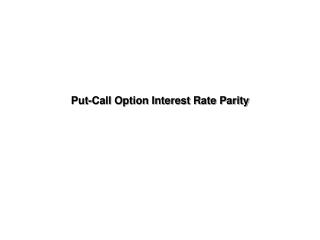Download PresentationPut-Call Option Interest Rate Parity

# Put-Call Option Interest Rate Parity - PowerPoint PPT PresentationDownload Presentation## Put-Call Option Interest Rate Parity

- - - - - - - - - - - - - - - - - - - - - - - - - - - E N D - - - - - - - - - - - - - - - - - - - - - - - - - - -
##### Presentation Transcript

1. Put-Call Option Interest Rate Parity

2. Objective Determine the international parity relationship between Call, Put, and Forward prices

3. Outline • Two arbitrage portfolios • Derivation of parity conditions • Exemplification

4. Two arbitrage portfolios Consider: C: the premium of a call option on the Sfr P: the premium of a put option on the Sfr X: the strike price of call and put options niSfr : Sfr nominal interest rate ni\$ : \$ nominal interest rate s = \$/Sfr : spot exchange rate f = forward rate

5. Two arbitrage portfoliose = \$/Sfr

6. Two arbitrage portfolioss = \$/Sfr

7. Two arbitrage portfolioss = \$/Sfr

8. Two arbitrage portfolioss = \$/Sfr

9. Two arbitrage portfolioss = \$/Sfr

10. Two arbitrage portfolioss = \$/Sfr

11. Parity conditions derived It follows that C = s0/(1+niSfr) - X/(1+ni\$) +P According to interest rate parity we know that s0/(1+niSfr) = f1/(1+ni\$) Hence, if we are in Canada, C = (f1 - X)/(1+ni\$) + P In general, C = (f1 - X)/(1+nih) + P

12. Exemplification e0 = C\$0.7143/Sfr ni\$ = 3.5% niSfr = 4.4% One-year forward = C\$0.70814/Sfr A call on the Sfr struck at C\$0.701/Sfr, expiring in one year sells at C\$0.035/Sfr A put on the Sfr struck at C\$0.701/Sfr, expiring in one year sells at C\$0.023/Sfr

13. Note C\$0.70814/C\$0.7143 = (1.035)/(1.044) IRP holds C\$0.035 > C\$(0.70814-0.701)/(1.035) + C\$0.023 Arbitrage opportunity

14. Another two arbitrage portfolios

15. Another two arbitrage portfolios

16. Another two arbitrage portfolios

17. Another two arbitrage portfolios

18. Analysis At expiration, the combined payoff from the two portfolios is always zero. However, buying the first portfolio and shorting the second one has produced an arbitrage profit of (C\$2,940- C\$2,511.25)=C\$428.75 up-front.